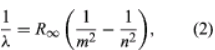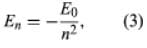Bohr’s theory of the atom

# Bohr’s theory of the atom - Notes | Study Modern Physics for IIT JAM - Physics

 1 Crore+ students have signed up on EduRev. Have you?

A major contribution to the subject was made by Niels Bohr of Denmark, who applied the quantum hypothesis to atomic spectra in 1913. The spectra of light emitted by gaseous atoms had been studied extensively since the mid-19th century. It was found that radiation from gaseous atoms at low pressure consists of a set of discrete wavelengths. This is quite unlike the radiation from a solid, which is distributed over a continuous range of wavelengths. The set of discrete wavelengths from gaseous atoms is known as a line spectrum, because the radiation (light) emitted consists of a series of sharp lines. The wavelengths of the lines are characteristic of the element and may form extremely complex patterns. The simplest spectra are those of atomic hydrogen and the alkali atoms (e.g., lithium, sodium, and potassium). For hydrogen, the wavelengths λ are given by the empirical formulawhere m and n are positive integers with n > m and R, known as the Rydberg constant, has the value 1.097373157 × 107 per metre. For a given value of m, the lines for varying n form a series. The lines for m = 1, the Lyman series, lie in the ultraviolet part of the spectrum; those for m = 2, the Balmer series, lie in the visible spectrum; and those for m = 3, the Paschen series, lie in the infrared.
Bohr started with a model suggested by the New Zealand-born British physicist Ernest Rutherford. The model was based on the experiments of Hans Geiger and Ernest Marsden, who in 1909 bombarded gold atoms with massive, fast-moving alpha particles; when some of these particles were deflected backward, Rutherford concluded that the atom has a massive, charged nucleus. In Rutherford’s model, the atom resembles a miniature solar system with the nucleus acting as the Sun and the electrons as the circulating planets. Bohr made three assumptions. First, he postulated that, in contrast to classical mechanics, where an infinite number of orbits is possible, an electron can be in only one of a discrete set of orbits, which he termed stationary states. Second, he postulated that the only orbits allowed are those for which the angular momentum of the electron is a whole number n times ℏ (ℏ = h/2π). Third, Bohr assumed that Newton’s laws of motion, so successful in calculating the paths of the planets around the Sun, also applied to electrons orbiting the nucleus. The force on the electron (the analogue of the gravitational force between the Sun and a planet) is the electrostatic attraction between the positively charged nucleus and the negatively charged electron. With these simple assumptions, he showed that the energy of the orbit has the formwhere E0 is a constant that may be expressed by a combination of the known constants e, me, and ℏ. While in a stationary state, the atom does not give off energy as light; however, when an electron makes a transition from a state with energy En to one with lower energy Em, a quantum of energy is radiated with frequency ν, given by the equation
hv = En - Em (4)
Inserting the expression for En into this equation and using the relation λν = c, where c is the speed of light, Bohr derived the formula for the wavelengths of the lines in the hydrogen spectrum, with the correct value of the Rydberg constant.
Bohr’s theory was a brilliant step forward. Its two most important features have survived in present-day quantum mechanics. They are (1) the existence of stationary, nonradiating states and (2) the relationship of radiation frequency to the energy difference between the initial and final states in a transition. Prior to Bohr, physicists had thought that the radiation frequency would be the same as the electron’s frequency of rotation in an orbit.

The document Bohr’s theory of the atom - Notes | Study Modern Physics for IIT JAM - Physics is a part of the Physics Course Modern Physics for IIT JAM.
All you need of Physics at this link: Physics

## Modern Physics for IIT JAM

54 videos|44 docs|15 tests
 Use Code STAYHOME200 and get INR 200 additional OFF

## Modern Physics for IIT JAM

54 videos|44 docs|15 tests

Track your progress, build streaks, highlight & save important lessons and more!

,

,

,

,

,

,

,

,

,

,

,

,

,

,

,

,

,

,

,

,

,

;